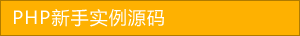﻿ 掌握PHP垃圾回收机制详解 - php高级应用 - PHP粉丝网

# 掌握PHP垃圾回收机制详解

##### 发布:smiling　来源: PHP粉丝网 　添加日期：2021-11-12 10:36:43　浏览: 评论：0

php的垃圾回收机制可以简单总结为 引用计数 写时复制 COW机制，本文主要和大家分享掌握php垃圾回收机制的知识，希望能帮助到大家。

1. <?php
2. //php zval变量容器
3. \$a = 1;
4. \$b = 1;
5. \$c = &\$a
6. \$d = \$b
7. \$e = range(0, 3);
8. xdebug_debug_zval('a');
9.  xdebug_debug_zval('b');
10. xdebug_debug_zval('c');
11.  xdebug_debug_zval('d');
12.  xdebug_debug_zval('e'); >>>>>>>>>>>>>>>>>>>>>>>>>>>>>>>>>>>>>>>>>>>>>>>>>

1. a:(refcount=2is_ref=1),int 1b:(refcount=2is_ref=0),int 1c:(refcount=2is_ref=1),int 1d:(refcount=2is_ref=0),int 1e:(refcount=1is_ref=0), array (size=40 => (refcount=1is_ref=0),int 0 1 => (refcount=1is_ref=0),int 1 2 => (refcount=1is_ref=0),int 2 3 => (refcount=1is_ref=0),int 3

1. <?php
2. //php zval变量容器
3. \$a = 1;
4. \$b = 1;
5. \$c = &\$a
6. \$d = \$b
7. \$e = range(0, 3);
8. xdebug_debug_zval('a');
9.  xdebug_debug_zval('b');
10. xdebug_debug_zval('c');
11.  xdebug_debug_zval('d');
12. xdebug_debug_zval('e'); >>>>>>>>>>>>>>>>>>>>>>>>>>>>>>>>>>>>>>>>>>>> 结果如下 a:(refcount=2, is_ref=1)int 1b:(refcount=0, is_ref=0)int 1c:(refcount=2, is_ref=1)int 1d:(refcount=0, is_ref=0)int 1e:(refcount=1, is_ref=0)array (size=4) 0 => (refcount=0, is_ref=0)int 0 1 => (refcount=0, is_ref=0)int 1 2 => (refcount=0, is_ref=0)int 2 3 => (refcount=0, is_ref=0)int 3

## 相关文章